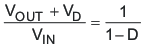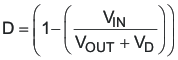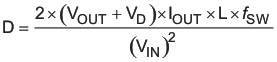SLUS772G MARCH   2008  – June 2020

PRODUCTION DATA.

1. Features
2. Applications
3. Description
1.     Device Images
4. Revision History
5. Pin Configuration and Functions
6. Specifications
7. Detailed Description
1. 7.1 Overview
2. 7.2 Functional Block Diagram
3. 7.3 Feature Description
4. 7.4 Device Functional Modes
8. Application and Implementation
1. 8.1 Application Information
2. 8.2 Typical Applications
1. 8.2.1 12-V to 24-V Nonsynchronous Boost Regulator
1. 8.2.1.1 Design Requirements
2. 8.2.1.2 Detailed Design Procedure
3. 8.2.1.3 Application Curves
2. 8.2.2 12-V Input, 700-mA LED Driver, Up to 35-V LED String
9. Power Supply Recommendations
10. 10Layout
11. 11Device and Documentation Support
1. 11.1 Device Support
2. 11.2 Documentation Support
6. 11.6 Electrostatic Discharge Caution
7. 11.7 Glossary
12. 12Mechanical, Packaging, and Orderable Information

• DGQ|10
• DRC|10
• DGQ|10
• DRC|10

7.3.4 Minimum On-Time and Off-Time Considerations

The TPS40210 has a minimum off-time of approximately 200 ns and a minimum on-time of 300 ns. These two constraints place limitations on the operating frequency that can be used for a given input-to-output conversion ratio. See Figure 2 for the maximum frequency that can be used for a given duty cycle.

The duty cycle at which the converter operates is dependent on the mode in which the converter is running. If the converter is running in discontinuous conduction mode, the duty cycle varies with changes to the load much more than it does when running in continuous conduction mode.

In continuous conduction mode, the duty cycle is related primarily to the input and output voltages.

Equation 10.Equation 11.In discontinuous mode, the duty cycle is a function of the load, input and output voltages, inductance, and switching frequency.

Equation 12.All converters using a diode as the freewheeling or catch component have a load current level at which they transition from discontinuous conduction to continuous conduction. This is the point where the inductor current just falls to zero. At higher load currents, the inductor current does not fall to zero but remains flowing in a positive direction and assumes a trapezoidal wave shape as opposed to a triangular wave shape. This load boundary between discontinuous conduction and continuous conduction can be found for a set of converter parameters as follows.

Equation 13.For loads higher than the result of Equation 13, the duty cycle is given by Equation 11 and for loads less that the results of Equation 13, the duty cycle is given Equation 12. For Equations 1 through 4, the variable definitions are as follows.

• VOUT is the output voltage of the converter in V
• VD is the forward conduction voltage drop across the rectifier or catch diode in V
• VIN is the input voltage to the converter in V
• IOUT is the output current of the converter in A
• L is the inductor value in H
• fSW is the switching frequency in Hz